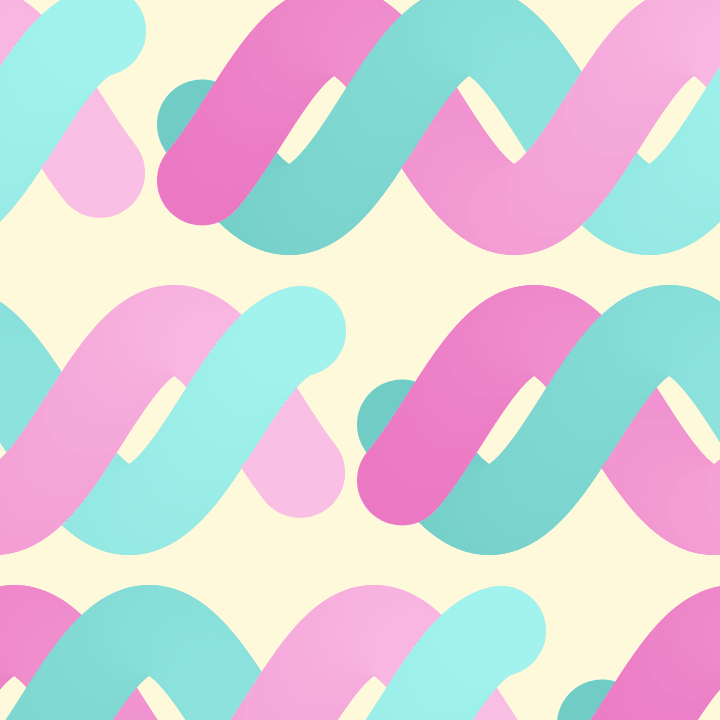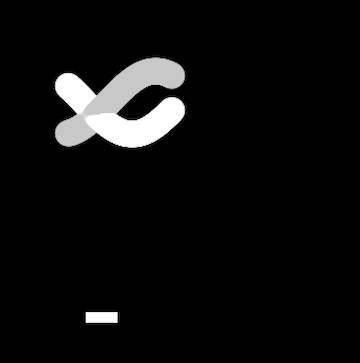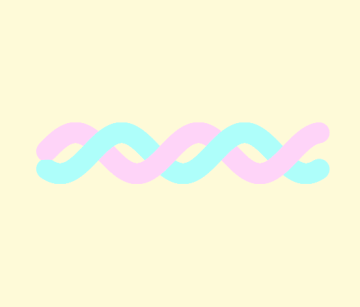# conye – AnimatedLoop

Below is a gif of the finished piece:Here are some sketches for this loop!I originally had imagined that it was a loop of very, very long weaving dogs chasing their own tail, but ended up changing plans. I think the current final result has nice simple forms that compliment their motions; however, in the future, I might end up adding dog heads/tails to them to see what the result looks like because that might make the loop more fun.

Here are some other sketches that I made originally! I actually did halfway implement both the cat sketch in the middle and the orchid sketch on the right, but wasn’t satisfied with either of them and had to move on.#### The process:

First, I worked on the weaving motion of the lines. It actually was harder than I imagined it would be because the lines would almost melt into each other where they intersected, so I had to draw the pieces of the “lines” (which are made up of ellipses) separately.

I ended up making the process unnecessarily difficult for myself by not making enough variables and helper functions, so my code was really difficult to debug, but at least I’ve learned my lesson- next time I will write cleaner code for the sake of sanity, and my time. Thank you to Golan for helping me debug my code! Adding the easing function that Golan really changed the whole feeling of the piece, and I’m really amazed by how useful Pattern Master is! I used the Double Quadratic Bezier function because I thought it had the right kind of up and down movement that would make the movement of the lines interesting to look at.

Below, to the left, is an early process image of when I was using the lower rectangle to test if I could correctly find where the intersections where. Next to it is after I got the weaving to work, but without the easing function and the gradient.The Processing code is below. For clarity, this is the non-template version, but I later made a few small edits to fit into Golan’s template.

```//global variables boolean overlap; int frame, offset; color[] bluegradient = new color; color[] redgradient = new color; int nFrames = 360; //number of frames   void setup() { frame = 0; size(720, 720); createGradients(); noStroke(); overlap = false; //overlap used to draw lines in the draw function offset = 135; //how much the second line is offset by }   void draw() { frame = (frameCount)% nFrames; //frame can be from 0 to 359 background(#FFFADA);   //this nested loop draws multiple squiggle pairs at different locations on the canvas for (int j = -2; j < 1; j++) { for (int k = 0; k < 3; k++) { //variables below control where this squiggle pair starts int startx = j * 720 + k*200 + 200; int starty = k * 300 + 120;   //i is a counter for the ellipses when we draw them, reset for every squiggle pair int i = 0;   //flip is used to weave boolean flip = false;   //the offset of 135 flips around the weaving at frame 22 and 310, //so I used the below to keep it from looking weird and glitchy if (frame < 22 || frame > 310) { flip = false; } else { flip = true; }   //this while loop is where the squiggles are drawn. Width is 620 right now. while (i < 620) { //overlap is true if the two lines are intersecting at this i value boolean overlap = Math.abs(helperSin(i, frame, 0) - helperSin(i, frame, offset)) < 100; //loop while it's not at the full width yet, and when they're not intersecting while (i < 620 && !overlap) { fill(redgradient[i/2]); ellipse(startx + (i + frame * 2), starty + helperSin(i, frame, offset), 90, 90); fill(bluegradient[i/2]); ellipse(startx + i + frame * 2, starty + helperSin(i, frame, 0), 90, 90); overlap = Math.abs(helperSin(i, frame, 0) - helperSin(i, frame, offset)) < 100; i+=3; //we do +3 to save processing power } //cap the length of this intersecting piece to be either 120 or 620 - i int cap = (i + 120 >= 620)? 620 - i : 120; int stopper = i + cap; //tells i where to stop based on how long cap is if (flip) {//if + else flip makes the intersection overlapping alternate while (i < stopper) { fill(redgradient[i/2]); ellipse(startx + (i) + frame * 2, starty + helperSin(i, frame, offset), 90, 90); i+=3; } i -= cap; while (i < stopper) { fill(bluegradient[i/2]); ellipse(startx + i + frame * 2, starty + helperSin(i, frame, 0), 90, 90); i+=3; } } else { while (i < stopper) { fill(bluegradient[i/2]); ellipse(startx + i + frame * 2, starty + helperSin(i, frame, 0), 90, 90); i+=3; } i -= cap; while (i < stopper) { fill(redgradient[i/2]); ellipse(startx + (i) + frame * 2, starty +helperSin(i, frame, offset), 90, 90); i+=3; } } flip = !flip; } } } }   //helper function that applies transformations to sin float helperSin(int i, int frame, int offset){ return 90 * sin(radians(i + DQB(frame) + offset)); }   //helper function for DoubleQuadraticBezier(...) float DQB(int x) { float x01 = (float)x/ (float)nFrames; return (float)(360 * function_DoubleQuadraticBezier (x01, 0.25, 0.75, 0.75, 0.25)); }   //Easing function from Golan Levin's Pattern Master float function_DoubleQuadraticBezier (float x, float a, float b, float c, float d) { //functionName = "Double Quadratic Bezier"; float xmid = (a + c)/2.0; float ymid = (b + d)/2.0; xmid = constrain (xmid, EPSILON, 1.0-EPSILON); ymid = constrain (ymid, EPSILON, 1.0-EPSILON); float y = 0; float om2a; float t; float xx; float aa; float bb; if (x <= xmid) { xx = x / xmid; aa = a / xmid; bb = b / ymid; om2a = 1.0 - 2.0*aa; if (om2a == 0) { om2a = EPSILON; } t = (sqrt(aa*aa + om2a*xx) - aa)/om2a; y = (1.0-2.0*bb)*(t*t) + (2*bb)*t; y *= ymid; } else { xx = (x - xmid)/(1.0-xmid); aa = (c - xmid)/(1.0-xmid); bb = (d - ymid)/(1.0-ymid); om2a = 1.0 - 2.0*aa; if (om2a == 0) { om2a = EPSILON; } t = (sqrt(aa*aa + om2a*xx) - aa)/om2a; y = (1.0-2.0*bb)*(t*t) + (2*bb)*t; y *= (1.0 - ymid); y += ymid; } return y; }   //creates those nice gradients void createGradients() { for (int i = 0; i < 400; i++) { int r = (int)( 113 + (64*(i/ 400.0))); int g = (int)( 204 + (51*(i/ 400.0))); int b = (int)( 198 + (52*(i/ 400.0))); bluegradient[i] = color(r, g, b); } for (int i = 0; i < 400; i++) { int r = (int)( 235 + (20*(i/ 400.0))); int g = (int)( 120 + (93*(i/ 400.0))); int b = (int)( 195 + (45*(i/ 400.0))); redgradient[i] = color(r, g, b); } }```